Difference between revisions of "Maximal ideal"

A maximal element in the partially ordered set of proper ideals of a corresponding algebraic structure. Maximal ideals play an essential role in ring theory. Every ring with identity has maximal left (also right and two-sided) ideals. The quotient module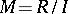ofregarded as a left (respectively, right)-module relative to a left (respectively, right) maximal idealis irreducible (cf. Irreducible module); a homomorphismofinto the field of endomorphisms ofis a representation of. The kernel of all such representations, that is, the set of elements of the ring which are mapped to zero by all representations, is called the Jacobson radical of; it coincides with the intersection of all maximal left (also, all right) ideals.
In the ring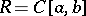of continuous real-valued functions on a closed interval, the set of functions vanishing at some fixed pointis a maximal ideal. Such ideals exhaust all maximal ideals of. This relation between the points of the interval and the maximal ideals has resulted in the construction of various theories for representing rings as rings of functions on a topological space.
The Zariski topology on the set of prime ideals (cf. Prime ideal)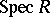of a ringhas weak separation properties (that is, there are non-closed points). A similar topology in the non-commutative case can be introduced on the setof primitive ideals (cf. Primitive ideal), which are the annihilators of irreducible-modules. The set of maximal ideals, and in the non-commutative case, of maximal primitive ideals, forms a subspace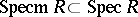which satisfies the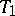-separation axiom.